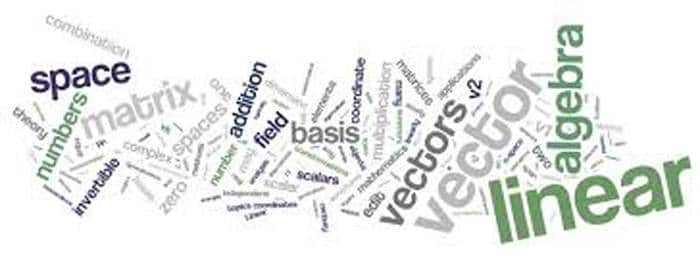Engineering Entrance Sample Papers

MAH CET Maths Practice Questions PaperThis Maharashtra CET Mathematics Practice sample paper is based on Mah CET Maths syllabus and consist 34 questions.

Ques: If cosh y = sec x, then the value of tanh2 (y/2) is
(a) tan2 (x/2)
(b) cot2 x/2
(c) sin2 (x/2)
(d) cos2 x/2
Ans:- (a)

Ques: P, Q, R and S have to give lectures to an audience. The organiser can arrange the order of their presentation in
(a) 4 ways
(b) 12 ways
(c) 256 ways
(d) 24 ways
Ans:- (d)

Ques: The number of values of c such that the straight line y = 4x + c touches the curve x2/4 + y2 = 1 is
(a) 0
(b) 1
(c) 2
(d) Infinite
Ans:- (c)

Ques: Equation of one of the sides of an isosceles right angled triangle whose hypotenuse is 3x + 4y = 4 and the opposite vertex of the hypotenuse is (2, 2), will be
(a) x – 7y + 12 = 0
(b) 7x + y – 12 = 0
(c) x – 7y + 16 = 0
(d) 7x + y + 16 = 0
Ans:- (a)

Ques: The vector b = 3j + 4k is to be written as the sum of a vector b1 parallel to a = I + j and a vector b2 perpendicular to a. Then b1 =
(a) 3/2 (i + j)
(b) 2/3 (I + j)
(c) ½ (I + j)
(d) 1/3 (i + j)
Ans:- (a)

Ques: A man of mass 80 kg. is travelling in a lift. The reaction between the floor of the lift and the man when the lift is ascending upwards at 4 m/sec2 is
(a) 1464.8 N
(b) 1784.8 N
(c) 1959.8 N
(d) 1104.8 N
Ans:- (d)

Ques: A pair of fair dice is rolled together till a sum of either 5 or 7 is obtained. Then the probability that 5 comes before 7 is
(a) 1/5
(b) 2/5
(c) 4/5
(d) None of theses
Ans:- (d)

Ques: G = {e, a, b, c} is an abelian group with e as identity element. The order of the other elements are
(a) 2, 2, 2
(b) 3, 3, 3
(c) 2, 2, 4
(d) 2, 3, 4
Ans:- (a)

Ques: The electronic components used in third generation of computers are
(a) Vacuum tubes
(b) Transistors
(c) Integrated circuit
(d) All of these
Ans:- (c)

Related: Maths Practice paper for UPSEAT

Ques: The triangle formed by the tangent to the curve f(x) = x2 + bx – b  at the point (1, 1) and the co-ordinate axes, lies in the first quadrant. If its area is 2 then the value of b is
(a) –1
(b) 3
(c) –3
(d) 1
Ans:- (c)

Ques: 49n + 16n – 1  is divisible by
(a) 3
(b) 19
(c) 64
(d) 29
Ans:- (c)

Ques: Two tangents PQ and PR drawn to the circle x2 + y2 – 2x – 4y – 20 = 0 from point P (16, 7). If the centre of the circle is C, then the area of quadrilateral PQCR will be
(a) 75 sq. units
(b) 150 sq. units
(c) 15 sq. units
(d) None of these
Ans:- (a)

Ques: The area of the triangle formed by the line 4x2 – 9xy – 9y2 = 0 and x = 2 is
(a) 2
(b) 3
(c) 10/3
(d) 20/3
Ans:- (c)

Ques: The differential equation for the family of curves x2 + y2 – 2ay = 0, where a is an arbitrary constant, is
(a) (x2 + y2)y’ = 2xy
(b) 2(x2 + y2)y’ = 2xy
(c) (x2 – y2)y’ = 2xy
(d) 2(x2 – y2)y’ = xy
Ans:- (c)

Ques: If one root of the equation f(x) = 0 is near to xo then the first approximation of this root as calculated by Newton-Raphson method is the abscissa of the point where the following straight line intersects the x-axis
(a) Normal to the curve y = f(x) at the point (x0, f(x0))
(b) Tangent to the curve y = f(x) at the point (x0, f(x0))
(c) The straight line through the point (x0, f(x0)) having the gradient 1/f’(x0)
(d) The ordinate through the point (x0, f(x0))
Ans:- (b)